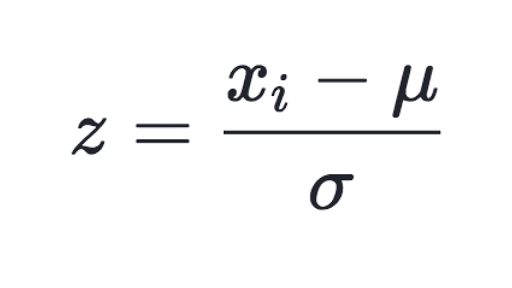# Standarization with pytorch

I am trying to solve a multiclass classification problem in which I have over 7000 pictures with labels. While preprocessing the data, I use a torchvision.Compose with Normalize() function included at the end. Said function performs the following operation for all the three channels:Which is convenient for many cases but what I need is to standarize my data, that is to say, make it with 0 mean and variance 1. I cannot seem to find an easy way to do this without using other libraries.

I am not providing code as my problem is not related to a specific error but more towards a general lack of knowledge that could not be solved by searching on this forum/Stack Overflow/torchvision documentation.

The mentioned approach will standardize your data, as you will subtract the mean (so that the output will have zero mean) and divide by the standard deviation (so that the output will have unit variance).

But which values should I give to the Normalize function?

Or, if no arguments are given, the function automatically standardizes the data? I have seen multiple uses of this function, most of the times gave Normalize([0.5,0.5,0.5],[0.5,0.5,0.5]). As tensors have values between 0 and 1 this gives would mean that the values would vary from -1 to 1. I have seen also more wacky approaches with different numbers for each of the parameters. I feel like I’m at the grasp of finally realizing how to solve my problem but I’m not quite there yet.

If you are using “ImageNet-like” images, you could try to use the calculated stats from the ImageNet dataset as used in our tutorials:

``````normalize = transforms.Normalize(mean=[0.485, 0.456, 0.406],
std=[0.229, 0.224, 0.225])
``````

You could of course calculate the mean and std of your training dataset manually and use these stats.
Note that the values are in the specified range, as you are usually calling `transforms.ToTensor()`, which returns a tensor in the range `[0, 1]`.

Even if your “real” stats are not exactly these numbers, the pretrained models will most likely work just fine.
However, if your data comes from a completely different domain, e.g. CT/MRI images, you might need to use your calculated stats.

1 Like